# The SEQDESIGN Procedure

### Applicable One-Sample Tests and Sample Size Computation

Subsections:

The SEQDESIGN procedure provides sample size computation for two one-sample tests: normal mean and binomial proportion. The required sample size depends on the variance of the response variable—that is, the sample proportion for a binomial proportion test.

In a typical clinical trial, a hypothesis is designed to reject, not accept, the null hypothesis to show the evidence for the alternative hypothesis. Thus, in most cases, the proportion under the alternative hypothesis is used to derive the required sample size. For a test of the binomial proportion, the REF=NULLPROP and REF=PROP options use proportions under the null and alternative hypotheses, respectively.

#### Test for a Normal Mean

The MODEL=ONESAMPLEMEAN option in the SAMPLESIZE statement derives the sample size required to test a normal mean by using the sample mean statistic for the null hypothesis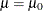. At stage k, the sample mean is computed as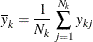where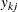is the value of the jth observation available in the kth stage and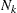is the cumulative sample size at stage k.

An equivalent hypothesis is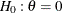, where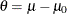.

The MLE statistic for,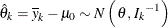where the information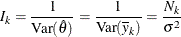is the inverse of the variance.

That is, the standardized statistic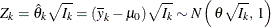Thus, to test the hypothesis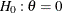against a two-sided alternative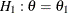,is rejected at stage k if the statistic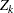is less than or equal to the lower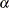boundary value or ifis greater than or equal to the upperboundary value at stage k.

If the varianceis unknown, the sample variance can be used if it is assumed that the sample variance is computed from a large sample such that the test statistic has an approximately normal distribution.

The maximum information is needed to derive the required sample size. If the maximum information is not specified or derived with the ALTREF= option in the procedure, the MEAN=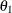option in the SAMPLESIZE statement is used to specify the alternative reference and thus to derive the maximum information.

In the SEQDESIGN procedure, the computed total sample size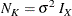where
is the maximum information andis the specified standard deviation. With an available maximum information, you can specify the MODEL=ONESAMPLEMEAN( STDDEV=) option in the SAMPLESIZE statement to compute the required total sample size and individual sample size at each stage. A procedure such as PROC MEANS can be used to derive a one-sample Z test for a normal mean.

#### Test for a Binomial Proportion

The MODEL=ONESAMPLEFREQ option in the SAMPLESIZE statement derives the sample size required to test a binomial proportion by using the null hypothesis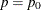, where p is the proportion of a binomial population. At stage k, the MLE for p is computed as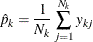whereis the value of the jth observation available in the kth stage andis the cumulative sample size at stage k.

An equivalent hypothesis is, where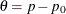. If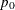is not close to 0 or 1, then for a large sample,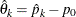has an approximately normal distribution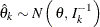where the information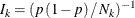is the inverse of the variance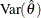.

Then the standardized statistic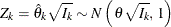In practice, the estimated sample proportion
at stage k can be used to derive the information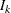and test statistic. Thus, to test the hypothesisagainst an upper alternative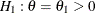,is rejected at stage k if the statisticis greater than or equal to the upperboundary at stage k.

The maximum informationis needed to derive the required sample size. If the maximum information is not specified or derived with the ALTREF= option in the procedure, the PROP= option in the SAMPLESIZE statement is used to specify the alternative reference and to derive the maximum information for the sample size calculation.

It is assumed that the sample size is sufficiently large such that the test statistic has an approximately normal distribution. With the hypotheses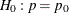and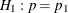, the SEQDESIGN procedure derives the total sample size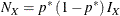where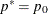if REF=NULLPROP is specified. Otherwise,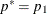.

If the PROP= option in the SAMPLESIZE statement is not specified, then the alternative referencederived in the SEQDESIGN procedure is used to compute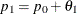.

The ALTREF= option in the PROC statement can be used to specify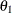. Otherwise, the PROP= option in the SAMPLESIZE statement must be specified.

For example, with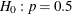,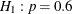, and REF=PROP (which is the default),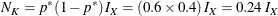You can specify the MODEL=ONESAMPLEFREQ option in the SAMPLESIZE statement to compute the required total sample size and individual sample size at each stage. A procedure such as PROC GENMOD with the default DIST=NORMAL option in the MODEL statement can be used to derive the Z test for a binomial proportion.